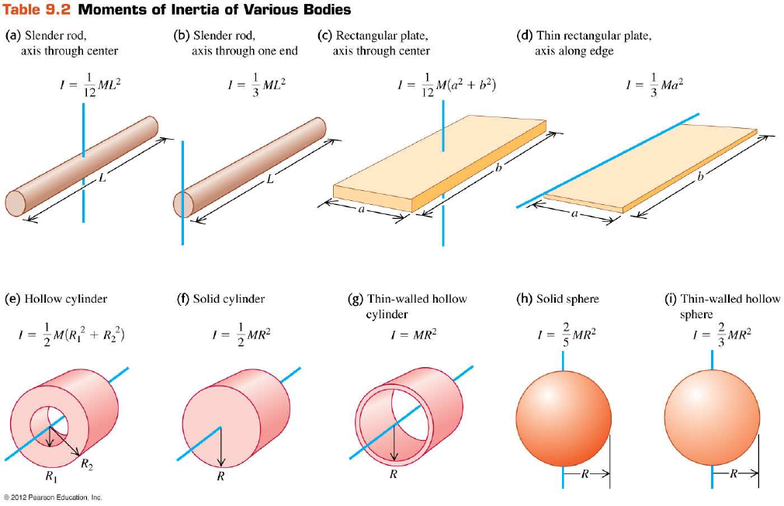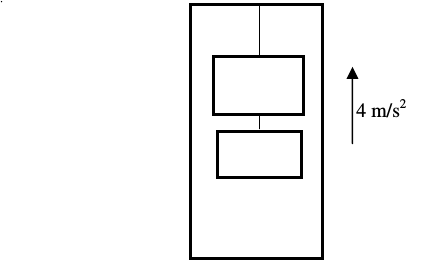Study Guides (390,000)
US (220,000)
U of M (5,000)
PHYSICS (100)
Final

# PHYSICS 140 Study Guide - Final Guide: Sound Intensity, Switch, Scantron CorporationExam

Department
Physics
Course Code
PHYSICS 140
Professor
Timothy McKay
Study Guide
Final

This preview shows pages 1-3. to view the full 15 pages of the document.Physics 140, Winter 2013
FINAL EXAM
April 26, 2013
Physics Department, University of Michigan
FORM 1
____________________________________________
INSTRUCTIONS AND INFORMATION
1. Fill in YOUR NAME, SECTION NUMBER and EXAM FORM NUMBER on the scantron.
This is a 120-minute, closed book exam. You may use four 3” x 5” cards on which
you have your favorite equations. You also may use a calculator but please do not
share calculators.
3. There are altogether 25 multiple choice questions. All questions are of equal value.
Equations that you may find useful:
s
f
= s
i
+ v
0
t + ½ a t
2
θ
f
= θ
in
+ ωt + ½ α t
2
Rod, axis through one end I =1/3ML
2
v
f
= v
0
+ at ω
f
= ω
0
+ α t Rod, axis through center: I = 1/12ML
2
a
= v
2
/r = rω
2
v
t
= r ω, a
t
= r α Solid sphere: I = 2/5 MR
2
F = ma = dp/dt τ = r × F = dL/dt=Iα
αα
α Hollow sphere: I = 2/3 MR
2
p = mv L = r ×
××
× p = Iω Solid cylinder: I = ½ MR
2
W = F.s F
k
= µ
k
N Hollow cylinder I = ½M(R
12
+R
22
)
F
x
= dU(x)/dx U
spring
= ½ kx
2
Hoop: I = MR
2
F =GM
1
M
2
/r
2
G = 6.67×10
-11
Nm
2
/kg
2
U = –GM
1
M
2
/r v
orb
= (GM/r)
1/2
W = K I = I
cm
+ Md
2
v
esc
= (2GM/R)
1/2
R
E
= 6.38×10
6
m
K + U = W
n.c.
K
rot
= ½ Iω
2
M
E
= 5.97×10
24
kg
in,2
21
2
in,1
21
21
f,1
v
mm
m2
v
mm
mm
v+
+
+
=
x(t) = A cos (ωt + φ) a
x
(t) = –ω
2
x
in.2
21
12
in,1
21
1
f,2
v
mm
mm
v
mm
m2
v+
+
+
=
ω=(k/m)
1/2
Simple pendulum: ω = (g/L)
1/2
Physical pendulum: ω = (mgd/I
pivot
)
1/2
Young’s modulus Y = (F
L
0
)/(AL) ρ
water
= 1000 kg/m
3
ρ
air
= 1.2 kg/m
3
p = p
o
+ ρgh p + ρgh + ρv
2
/2 = const.
1 atm = 1.013×10
5
Pa = 1.013 bar = 14.7 lb/in
2
1 mm Hg = 1 torr = 133.3 Pa
y(x,t) = Acos(kx -ωt) k = 2π/λ ω = 2πf = 2π/T v = (F/µ)
1/2
P(x,t) = µvω
2
A
2
sin
2
(kx - ωt) Beat frequency f
b
= f
1
-f
2
P
aver
= µvω
2
A
2
/2 f
L
= (v ± v
L
)/(v
v
S
) f
S
Sound intensity level in dB: β=10 log
10
(I/I
0
) Volume of sphere V = (4/3)πR
3

Only pages 1-3 are available for preview. Some parts have been intentionally blurred.Only pages 1-3 are available for preview. Some parts have been intentionally blurred.Physics 140, Winter 2013, Final Exam
Problem 1:
Two blocks, with masses 10 kg and 5 kg, are connected by light strings and attached to
the ceiling of an elevator as shown in figure. The elevator accelerates upward with 4
m/s
2
. What is the tension in the string attached to the ceiling of the elevator?
A. 69 N
B. 118 N
C. 138 N
D. 187 N
E. 207 N
Problem 2:
Which equation describes a plane wave travelling in the direction opposite to the x axis?
A. cos + 
B. cos + 
C. coscos
D. cos − 
E. cos + 
4 m/s
2
T ?
10 kg
5 kg